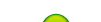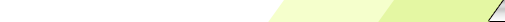Friday, 2020-02-28BioInfo Pakistan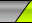Home | Sign Up | Log In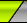Welcome Guest | RSS
Section categories
 Related Subjects This category includes brief overview of all related subjects. Defining BioInformatics In this section we tried to briefly explain what bioinformatics is ? Unviersities This contains information about universities that are offering bioinformatics degree programs. Resources Contains information about bioinformatics resources including databases, tools and techniques. Algorithms This category includes some of the basic algorithms that are usually used by bioinformaticians.
 Our poll Pakistani Student Yes No Total of answers: 0
 Chat Box Only authorized users can post messages
 StatisticsTotal online: 1 Guests: 1 Users: 0
Home » 2011 » August » 15 » Hirschberg's Algorithm
11:59 AM
Hirschberg's Algorithm

 Hirschberg's Algorithm1. Description:   Hirschberg's algorithm, named after its inventor, Dan Hirschberg, is a dynamic programming algorithm that finds the least cost sequence alignment between two strings, where cost is measured as Levenshtein edit distance, defined to be the sum of the costs of insertions, replacements, deletions, and null actions needed to change one string to the other. Hirschberg's algorithm is simply described as a divide and conquer version of the Needleman-Wunsch algorithm.   Hirschberg's algorithm is commonly used in computational biology to find maximal global alignments of DNA and protein sequences.2. Algorithm Information:   Hirschberg's algorithm is a generally applicable algorithm for finding an optimal sequence alignment. BLAST and FASTA are suboptimal heuristics. If x and y are strings, where length(x) = n and length(y) = m, the Needleman-Wunsch algorithm finds an optimal alignment in O(nm) time, using O(nm) space. Hirschberg's algorithm is a clever modification of the Needleman-Wunsch Algorithm which still takes O(nm) time, but needs only O(min{n,m}) space.One application of the algorithm is finding sequence alignments of DNA or protein sequences. It is also a space-efficient way to calculate the longest common subsequence between two sets of data such as with the common diff tool.3. Computation of the Levenshtein edit distance in linear space   The edit distance Edit(x,y) is the least cost of changing x into y by using the operations Insert, Substitute, and Delete, where the cost of each kind of operation is given. We write Ins(a) for the cost of inserting a symbol a into a string, Sub(a,b) for the cost of substituting a symbol b for a in a string, and Del(a) for the cost of deleting a symbol a from a string. The "standard" choice of those costs is Ins(a) = Del(a) = 1 for each symbol a, Sub(a,a) = 0, and Sub(a,b) = 1 if a is not equal to b. The Needleman-Wunsch algorithm computes Edit(x,y) by computing Edit(Prefix[x,i],Prefix[y,j]) for all i and j dynamically, where Prefix[x,i] denotes the prefix of x of length i. That algorithm requires O(nm) time and O(nm) space, where n = length(x) and m = length(y). 4. Algorithm Organization   To understand Hirschberg's algorithm, it is important to first understand that edit distances can be computed using linear space.   What we call the "forward subprogram" computes the values of Edit(Prefix[x,i],Prefix[y,j]) for all i and j, just as the Needleman-Wunsch and returns the array {Edit(x,Prefix[y,j])}0 ≤ j ≤ m. The "backward subprogram" is similar, except that the dynamic program is done in the opposite direction, i.e., starting from the right ends of the strings. It returns the array {Edit(x,Suffix[y,j])}0 ≤ j ≤ m, where Suffix[y,j] is the suffix of y of length j.5. The Algorithm:Compute V(A, B) while saving the values of the  -th row. Denote D(A,B) as the Forward Matrix F.Compute V(Ar, Br) while saving the  -th row. Denote D(Ar, Br) as the Backward Matrix B.Find the column k* so that the crossing point (  , k*) satisfies: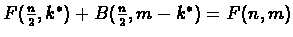Now that k* is found, recursively partition the problem to two sub problems:Find the path from (0,0) to (  , k*). Find the path from (n, m) to (  , m - k*).pseudocode
Category: Algorithms | Views: 1249 | Added by: Ansari | Rating: 0.0/0